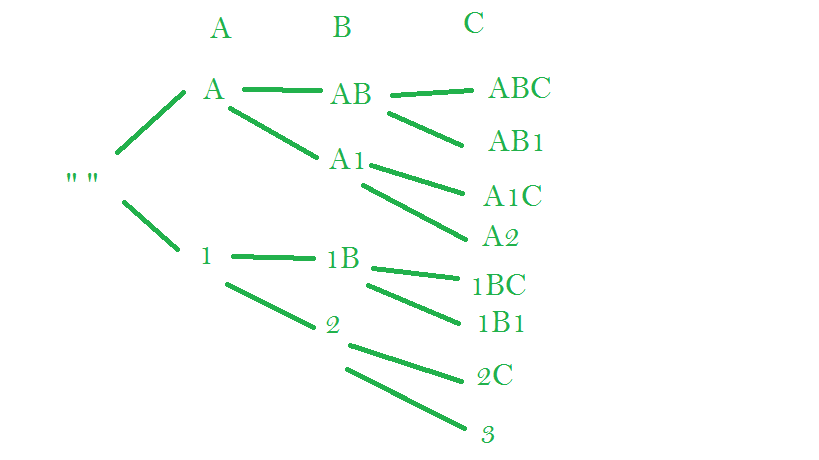Related Articles
Alphanumeric Abbreviations of a String
• Difficulty Level : Hard
• Last Updated : 29 Jul, 2017

Given a string of characters of length less than 10. We need to print all the alpha-numeric abbreviation of the string.

The alpha-numeric abbreviation is in the form of characters mixed with the digits which is equal to the number of skipped characters of a selected substring. So, whenever a substring of characters is skipped, you have to replace it with the digit denoting the number of characters in the substring. There may be any number of skipped substrings of a string. No two substrings should be adjacent to each other. Hence, no two digits are adjacent in the result. For a clearer idea, see the example.

Examples:

```Input : ANKS
Output :
ANKS (nothing is replaced)
ANK1 (S is replaced)
AN1S (K is replaced)
AN2  (KS is replaced)
A1KS (N is replaced)
A1K1 (N and S are replaced)
A2S (NK is replaced)
A3 (NKS is replaced)
1NKS (A is replaced)
1NK1 (A and S are replaced)
1N1S (A and N is replaced)
1N2 (A and KS are replaced)
2KS (AN is replaced)
2K1 (AN and S is replaced)
3S (ANK is replaced)
4 (ANKS is replaced)

Input : ABC
Output :
ABC
AB1
A1C
A2
1BC
1B1
2C
3
Note: 11C is not valid because no two digits should be adjacent,
2C is the correct one because AB is a substring, not A and B individually

```

## Recommended: Please try your approach on {IDE} first, before moving on to the solution.

The idea is to start with empty string. At every step, we have two choices.

1. Consider character as it is.
2. Add character to count. If there is no count, then use 1.You can see how each character can either add up to the result as a character or as a digit. This further gives rise to 2^n abbreviations at the end where n is the length of string.

 `// C++ program to print all Alpha-Numeric Abbreviations``// of a String``#include ``using` `namespace` `std;`` ` `// Recursive function to print the valid combinations``// s is string, st is resultant string``void` `printCompRec(``const` `string& s, ``int` `index,``                  ``int` `max_index, string st)``{``    ``// if the end of the string is reached``    ``if` `(index == max_index) {``        ``cout << st << ``"\n"``;``        ``return``;``    ``}`` ` `    ``// push the current character to result``    ``st.push_back(s[index]);`` ` `    ``// recur for the next [Using Char]``    ``printCompRec(s, index + 1, max_index, st);`` ` `    ``// remove the character from result``    ``st.pop_back();`` ` `    ``// set count of digits to 1``    ``int` `count = 1;`` ` `    ``// addition the adjacent digits``    ``if` `(!st.empty()) {`` ` `        ``if` `(``isdigit``(st.back())) {`` ` `            ``// get the digit and increase the count``            ``count += (``int``)(st.back() - ``'0'``);`` ` `            ``// remove the adjacent digit``            ``st.pop_back();``        ``}``    ``}`` ` `    ``// change count to a character``    ``char` `to_print = (``char``)(count + ``'0'``);`` ` `    ``// add the character to result``    ``st.push_back(to_print);`` ` `    ``// recur for this again [Using Count]``    ``printCompRec(s, index + 1, max_index, st);``}`` ` `// Wrapper function``void` `printComb(std::string s)``{``    ``// if the string is empty``    ``if` `(!s.length())``        ``return``;`` ` `    ``// Stores result strings one one by one``    ``string st;`` ` `    ``printCompRec(s, 0, s.length(), st);``}`` ` `// driver function``int` `main()``{``    ``string str = ``"GFG"``;``    ``printComb(str);``    ``return` `0;``}`

Output:

```GFG
GF1
G1G
G2
1FG
1F1
2G
3

```

Source: CareerCup

This article is contributed by Aditya Nihal Kumar Singh. If you like GeeksforGeeks and would like to contribute, you can also write an article using contribute.geeksforgeeks.org or mail your article to contribute@geeksforgeeks.org. See your article appearing on the GeeksforGeeks main page and help other Geeks.